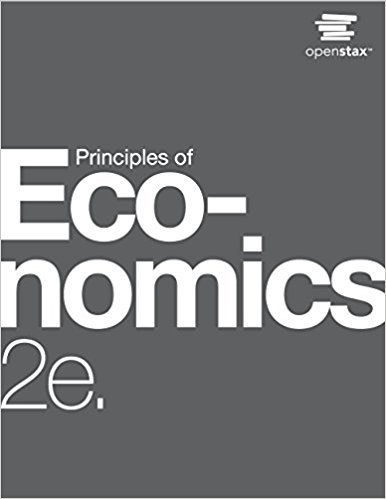×

×

# Solutions for Chapter 22: Inflation## Full solutions for Principles of Economics | 2nd Edition

ISBN: 9781947172364Solutions for Chapter 22: Inflation

Solutions for Chapter 22
4 5 0 261 Reviews
24
4
##### ISBN: 9781947172364

This textbook survival guide was created for the textbook: Principles of Economics, edition: 2. Chapter 22: Inflation includes 37 full step-by-step solutions. Principles of Economics was written by and is associated to the ISBN: 9781947172364. This expansive textbook survival guide covers the following chapters and their solutions. Since 37 problems in chapter 22: Inflation have been answered, more than 45631 students have viewed full step-by-step solutions from this chapter.

Key Business Terms and definitions covered in this textbook
• Arrow’s impossibility theorem

a mathematical result showing that, under certain assumed conditions, there is no scheme for aggregating individual preferences into a valid set of social preferences

• complements

two goods for which an increase in the price of one leads to a decrease in the demand for the other

• complements

two goods for which an increase in the price of one leads to a decrease in the demand for the other

• efficient scale

the quantity of output that minimizes average total cost

• equilibrium

a situation in which the market price has reached the level at which quantity supplied equals quantity demanded

• equilibrium quantity

the quantity supplied and the quantity demanded at the equilibrium price

• Federal Reserve (Fed)

the central bank of the United States

• fiat money

money without intrinsic value that is used as money because of government decree

• money multiplier

the amount of money the banking system generates with each dollar of reserves

• normative statements

claims that attempt to prescribe how the world should be

• price discrimination

the business practice of selling the same good at different prices to different customers

• price elasticity of demand

a measure of how much the quantity demanded of a good responds to a change in the price of that good, computed as the percentage change in quantity demanded divided by the percentage change in price

• proportional tax

a tax for which highincome and low-income taxpayers pay the same fraction of income

• rivalry in consumption

the property of a good whereby one person’s use diminishes other people’s use

• screening

an action taken by an uninformed party to induce an informed party to reveal information

• strike

the organized withdrawal of labor from a firm by a union

• sunk cost

a cost that has already been committed and cannot be recovered

• supply schedule

a table that shows the relationship between the price of a good and the quantity supplied

• surplus

a situation in which quantity supplied is greater than quantity demanded

• transaction costs

the costs that parties incur in the process of agreeing to and following through on a bargain